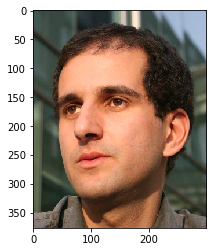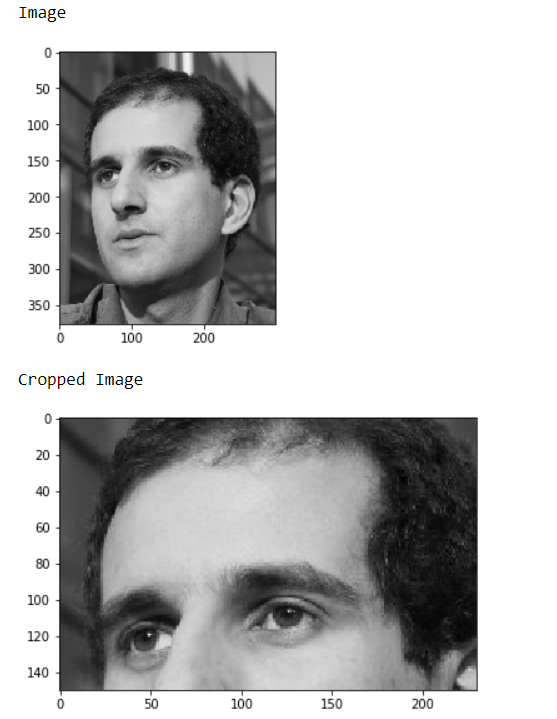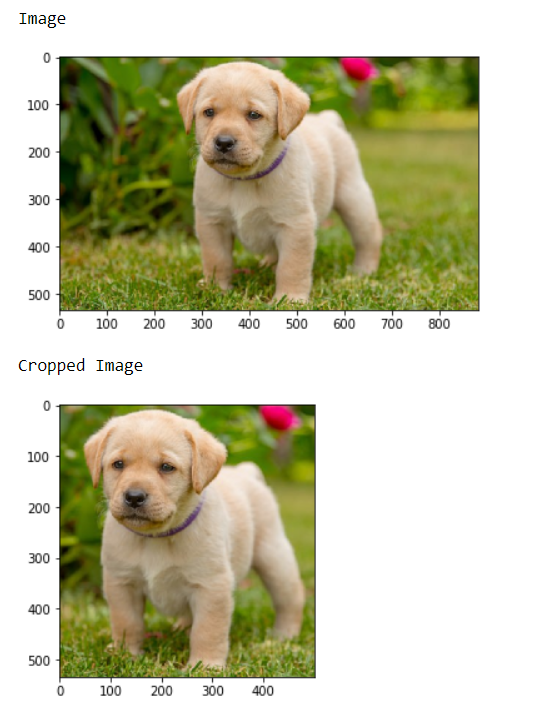# Mahotas – Cropping Image

In this article we will see how we can crop the image in mahotas. Cropping is easily done simply by slicing the correct part out of the array, here array is the image object which is numpy.ndarray.

In this tutorial we will use “luispedro” image, below is the command to load it.

`mahotas.demos.load('luispedro')`

Below is the luispedro imageIn order to do this we will take the image object which is numpy.ndarray and filter it with the help of indexing, below is the command to do this

`image = image[i1:i2, j1:j2]`

Example 1:

 `# importing required libraries ` `import` `numpy as np ` `import` `mahotas ` `import` `mahotas.demos ` `from` `mahotas.thresholding ``import` `soft_threshold ` `from` `matplotlib ``import` `pyplot as plt ` `from` `os ``import` `path ` ` `  `# loading image as grey ` `f ``=` `mahotas.demos.load(``'luispedro'``, as_grey ``=` `True``) ` ` `  `# making plt grey ` `plt.gray() ` ` `  `# showing image ` `print``(``"Image"``) ` `plt.imshow(f) ` `plt.show() ` ` `  `# cropping image ` `f ``=` `f[``50``:``200``, ``20``: ``250``] ` ` `  ` `  `# Show the image ` `print``(``"Cropped Image"``) ` `plt.imshow(f) ` `plt.show() `

Output :Example 2:

 `# importing required libraries ` `import` `mahotas ` `import` `numpy as np ` `from` `pylab ``import` `imshow, show ` `import` `os ` ` `  ` `  `# loading iamge ` `img ``=` `mahotas.imread(``'dog_image.png'``) ` ` `  `# showing image ` `print``(``"Image"``) ` `imshow(img) ` `show() ` ` `  `     `  ` `  `# cropping image ` `img ``=` `img[:, ``200``:``700``] ` ` `  ` `  `# showing the image ` `print``(``"Cropped Image"``) ` `imshow(img) ` `show() `

Output :My Personal Notes arrow_drop_upCheck out this Author's contributed articles.

If you like GeeksforGeeks and would like to contribute, you can also write an article using contribute.geeksforgeeks.org or mail your article to contribute@geeksforgeeks.org. See your article appearing on the GeeksforGeeks main page and help other Geeks.

Please Improve this article if you find anything incorrect by clicking on the "Improve Article" button below.

Article Tags :

Be the First to upvote.

Please write to us at contribute@geeksforgeeks.org to report any issue with the above content.